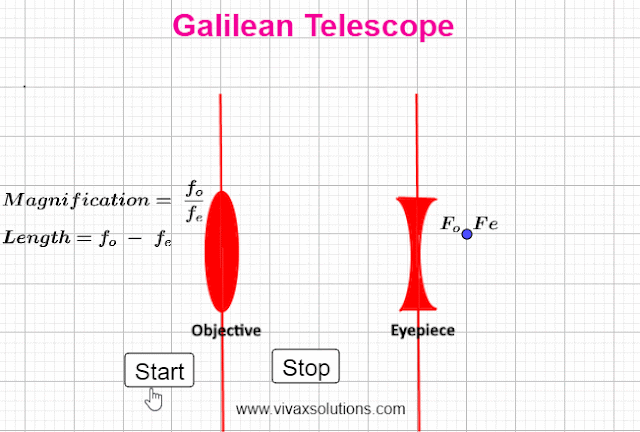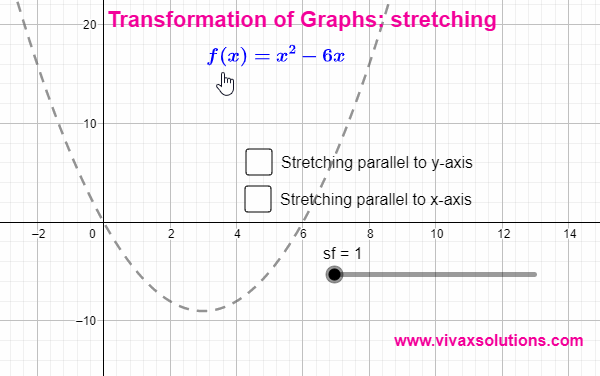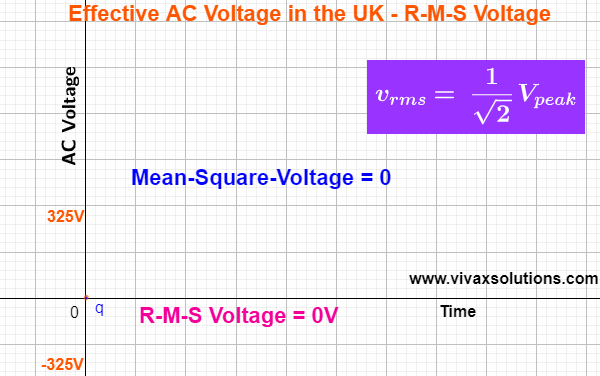## Posts

Showing posts from February 13, 2022

### Galilean TelescopeThe Galilean Telescope was invented by the legendary Italian physicist, Galileo Galilei in 1609. It is a simple telescope consisting of a convex lens and a concave lens; they act as the objective and eyepiece respectively. With the aid of his invention, Galileo managed to look at  J upiter’s four largest satellites, spots on the Sun, phases of Venus, and hills and valleys on the Moon.     It's final image, unlike in astronomical telescopes, is erect. As a result it can be used to look at objects on the Earth too. If the focal length of the two lenses are f o and f e respectively, Magnification in normal adjustment = f o / f e Length of the telescope  = f o - f e One of the significant disadvantage, however, is the small field view that in turn makes it difficult to view the images. Unfortunately, the relatively shorter length cannot compensate for this drawback.  For interactive practice, you can use the following applet:

### Galileo Galilei in a Jigsaw Puzzle - interactive playThe above jigsaw puzzle is of a famous physicist who was born in the month of February. He was instrumental in turning the astronomy upside down with his discoveries and theories despite being at odds with a powerful religious establishment at that time. You can find out who he is by playing with the following:

### Transformation of Graphs - stretchingThe tranformations of graphs are aimed at: GCSE IGCSE GCE -OL GCE - AL AS A Level High School T he above animation shows the strtching of a graph parallel to y-axis and x-axis. f(x) → af(x) is stretching parallel to y-axis by a factor a. E.g. f(x) = x² - 2x → 2f(x) = 2(x² - 2x) f(x) → f(ax) is stretching parallel to x-axis by a factor 1/a. E.g. f(x) = x² - 2x → f(2x) = (2x)² - 2(2x) You can practise the above interactively with the following applet:

### Root-Mean-Square Voltage - r-m-s voltage of ACRoot-Mean-Square Voltage in the UK The root-mean-square value of a function, f(t), is calculated as follows: Integrate the square of the function in the given range of the variable - from a to b in this case. Find the mean value of the above integral, dividing by the range of the variable - (b - a) in this case. Find the square root of the value that you get in step 2). The AC voltage is a sine curve and it changes with time as follows: v = 325 sin(t), where the peak value in the UK is about 325V. The calculation is as follows: The r.m.s value of the AC gives the same power given by an equal DC through the same resistor. E.g. If the resistor in question is 5 Ω, peak voltage and r-m-s voltage are 325V and 230V respectively,  Power = V 2 /R Power = 230 2 /5, not 325 2 /5, which is the same as under 230V, DC through  5 Ω resistor . For interactive practise, here is the applet: